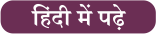# Triangles: Notes and Questions

Table of Contents

Mathematics is one of the most important that is asked in the government recruitment exams. Generally, there are questions asked related to basic concepts and formulas of trigonometry. To let you make the most of the Mathematics section, we are providing important notes related to triangle. Also, many central and state exams are nearby with bunches of posts for the interested candidates in which Mathematics is a major part. We have covered important notes and questions focusing on these prestigious exams. We wish you all the best of luck to come over the fear of the Mathematics section.

### Triangle

1). Centroid : [Intersecting Point of Medians ]/ केन्द्रक: माध्य का अंतर्बिंदु
2). Incenter → [Intersecting Point of Internal angle bisector] / अन्तः केन्द्र → [आंतरिक कोण द्विभाजक का प्रतिछेदन बिंदु ]
3).Circumcenter: → [Intersecting point of Perpendicular bisector] / परिकेंद्र :  [लम्बवत द्विभाजक का प्रतिछेदन बिंदु]
4). Orthocenter: → [Intersecting Point of Altitudes] / लंब केंद्र: → [ऊंचाई का प्रतिछेदन बिंदु]
Important Points:
(a)  Orthocenter of right angled triangle ⇒ at right angled vertex(समकोण त्रिभुज का लांब केंद्र ⇒   समकोण के शीर्ष पर )
(b)  Circumcenter of right angled triangle ⇒ Mid-point of Hypotenuse / समकोण का परिकेंद्र ⇒ मध्य बिंदु का कर्ण
(c)  Distance b/w incenter & circumcenter of a triangle / एक त्रिकोण के आतंरिक और परित्रिज्या के मध्य की दूरी
(d)  In Equilateral triangle / समबाहु त्रिभुज में,

### Questions

Q. Find the incentre of the triangle the coordinates of whose vertices are given by A(x1, y1), B(x2, y2), C(x3, y3).

Solution:

By geometry, we know that BD/DC = AB/AC (since AD bisects ÐA).
The lengths of the sides AB, BC and AC are c, a and b respectively, then BD/DC = AB/AC = c/b.
Coordinates of D are (bx2+cx3/b+c, by2+cy3/b+c)
IB bisects DB. Hence ID/IA = BD/BA = (ac/b+c)/c = a/c+b.
Let the coordinates of I be (x, y).
Then x = ax1+bx2+cx3/a+b+c, y = ay1+by2+cy3/a+b+c.

Q. If (0, 1), (1, 1) and (1, 0) are middle points of the sides of a triangle, find its incentre.

Solution:

Let A(x1, y1), B(x2, y2) and C(x3, y3)be teh vertices of a triangle.
x1 + x2 = 0, x2 + x= 0, x3 + x1 = 0
y1 + y2 = 0, y2 + y3 = 0, y3 + y1 = 0.
Solving these equations, we get A(0, 0), B(0, 2) and C(2, 0).
Now, a = BC = 2√ 2, b = CA = 2 and c = AB = 2.
Thus, incentre of the triangle ABC is (2-√ 2, 2-√ 2).

Q. If midpoints of the sides of a triangle are (0, 4), (6, 4) and (6, 0), then find the vertices of triangle, centroid and circumcentre of triangle.Solution:

Let points A (x1, y1), B (x2, y2) and C (x3, y3) be vertices of ΔABC.
x1 + x3 = 0 , y1 + y3 = 8
x2 + x3 = 12 , y2 + y3 = 8
x1 + x2 = 12, y1 + y2 = 0
Solving we get A (0, 0), B (12, 0) and C (0, 8)
Hence ΔABC is right angled triangle. ∠A = π/2
Circumcentre is midpoint of hypotenuse which is (6, 4) itself and centroid
(x1+x2+x3)/3 , (y1+y2+y3)/3 = (4 , 8/3)×

## Download success!

Thanks for downloading the guide. For similar guides, free study material, quizzes, videos and job alerts you can download the Adda247 app from play store.

Thank You, Your details have been submitted we will get back to you.

## Leave a commentJoin India's largest learning destination

What You Will get ?

•Job Alerts
•Daily Quizes
•Subject-Wise Quizes
•Current Affairs
•Previous year question papers
•Doubt Solving session

## Login

OR

Forgot Password?Join India's largest learning destination

What You Will get ?

•Job Alerts
•Daily Quizes
•Subject-Wise Quizes
•Current Affairs
•Previous year question papers
•Doubt Solving session

## Sign Up

ORJoin India's largest learning destination

What You Will get ?

•Job Alerts
•Daily Quizes
•Subject-Wise Quizes
•Current Affairs
•Previous year question papers
•Doubt Solving session

## Forgot Password

Enter the email address associated with your account, and we'll email you an OTP to verify it's you.Join India's largest learning destination

What You Will get ?

•Job Alerts
•Daily Quizes
•Subject-Wise Quizes
•Current Affairs
•Previous year question papers
•Doubt Solving session

## Enter OTP

Please enter the OTP sent to
/6

Did not recive OTP?

Resend in 60sJoin India's largest learning destination

What You Will get ?

•Job Alerts
•Daily Quizes
•Subject-Wise Quizes
•Current Affairs
•Previous year question papers
•Doubt Solving session

## Change PasswordJoin India's largest learning destination

What You Will get ?

•Job Alerts
•Daily Quizes
•Subject-Wise Quizes
•Current Affairs
•Previous year question papers
•Doubt Solving session

## Almost there

Please enter your phone no. to proceed
+91Join India's largest learning destination

What You Will get ?

•Job Alerts
•Daily Quizes
•Subject-Wise Quizes
•Current Affairs
•Previous year question papers
•Doubt Solving session

## Enter OTP

Please enter the OTP sent to Edit Number

Did not recive OTP?

Resend 60

## By skipping this step you will not recieve any free content avalaible on adda247, also you will miss onto notification and job alerts

Are you sure you want to skip this step?

## By skipping this step you will not recieve any free content avalaible on adda247, also you will miss onto notification and job alerts

Are you sure you want to skip this step?